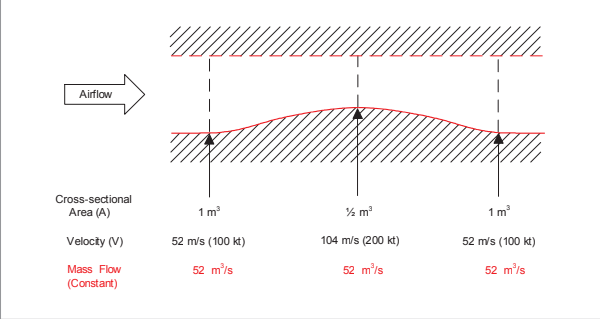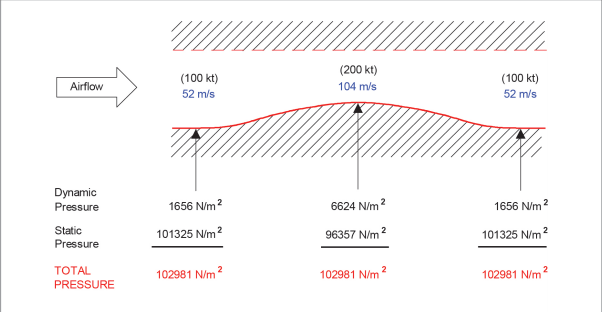Home Aviation Why The Airflow Has A Greater Velocity At The Upper Surface Of An Aerofoil?

# Why The Airflow Has A Greater Velocity At The Upper Surface Of An Aerofoil?

Whenever we ask someone how an aircraft produces lift they are able to answer based on Bernoulli Principle(BP); the airflow above the aerofoil has a higher velocity whereas the airflow on the lower surface of the aerofoil has a lower velocity. Based on BP the higher the velocity the lower the pressure therefore the difference in air pressure produces lift.

When a follow-up question is asked why the airflow on the upper surface is great, a majority of us are unable to answer. So today I will be splitting on Why & How the airflow has a greater velocity on the upper surface.

### This is a typical Cambered Aerofoil (Asymmetrical Aerofoil):##### Just for your information. Cambered aerofoils are not used in most modern aircraft, Symmetrical and Supercritical aerofoils are used.

To start off, Aerofoil basically uses two principle in producing lift:

1. The Principle Of Continuity
2. Bernoulli Principle

### 1.The Principle Of ContinuityThe air mass flow, or mass per unit time, through the tube will be the product of the cross-sectional area (A), the airflow velocity (V) and the air density (ρ).

Mass flow will remain a constant value at all points along the tube. The Equation of Continuity is:

A × V × ρ = Constant

For An Example, The Mass Flow throughout Point 1, 2 and 3 is the same.Which means that the flow of mass is constant throughout every part of the tube. When a same amount of volume passes through a narrower tube the velocity increases whereas when it travels through a wider tube the velocity is lower.

### 2. Bernoulli Principle:

Bernoulli’s Theorem
“In the steady flow of an ideal fluid the sum of the pressure energy and the kinetic energy remains constant”.
Note: An ideal fluid is both incompressible and has no viscosity.
This statement can be expressed as: Pressure + Kinetic energy = Constant

The upper surface of an aerofoil acts like the convergent duct while the lower surface acts like the divergent duct.Because the velocity of air at the throat has doubled, its dynamic pressure has risen by a value of four, and the static pressure has decreased. The significant point is that:
Static Pressure + Dynamic Pressure is a constant.

This constant can be referred to either as:

TOTAL PRESSURE, STAGNATION PRESSURE or PITOT PRESSURE.

It can be seen that flow velocity is dependent on the shape of the object over which it flows.And from Bernoulli’s theorem, it is evident that an increase in velocity will cause a decrease in static pressure, and vice versa.

As the airflow converges the Velocity increases, Pressure Drops

As the airflow diverges the Velocity decreases, Pressure Increases

Convergent = Upper Surface= Upwash

Divergent = Lower Surface=Downwash

The part where the airflow splits to upwash and downwash is known as the stagnation point. At this point the velocity of airflow is = 0#### Conclusion:

Continuity:
• Air accelerates when the cross-sectional area of a streamline flow is reduced.
• Air decelerates when the cross-sectional area increases again.

Bernoulli:
• If a streamline flow of air accelerates, its kinetic energy will increase and its static pressure
will decrease.
• When air decelerates, the kinetic energy will decrease and the static pressure will increase
again.

Wrong Theory: